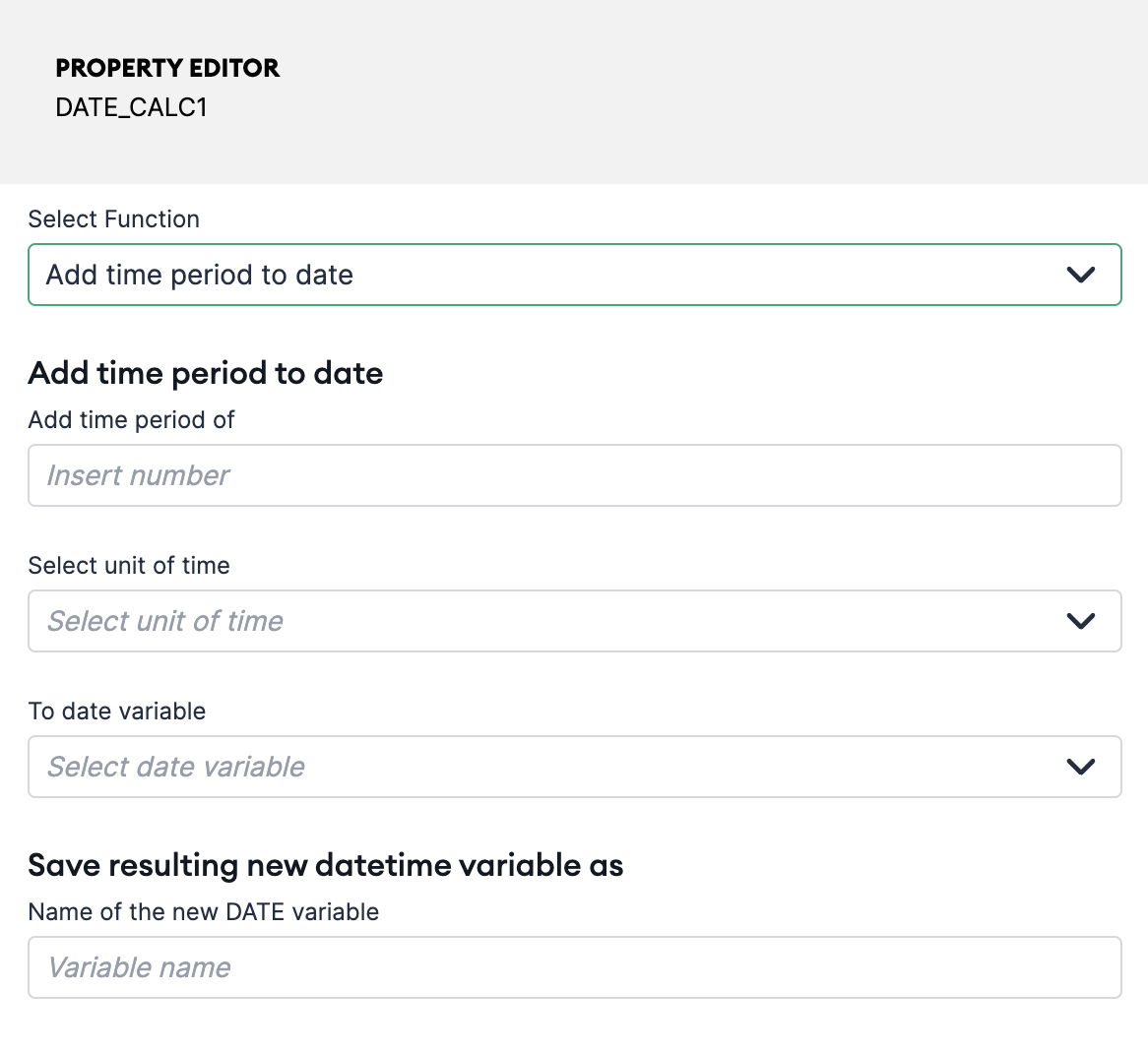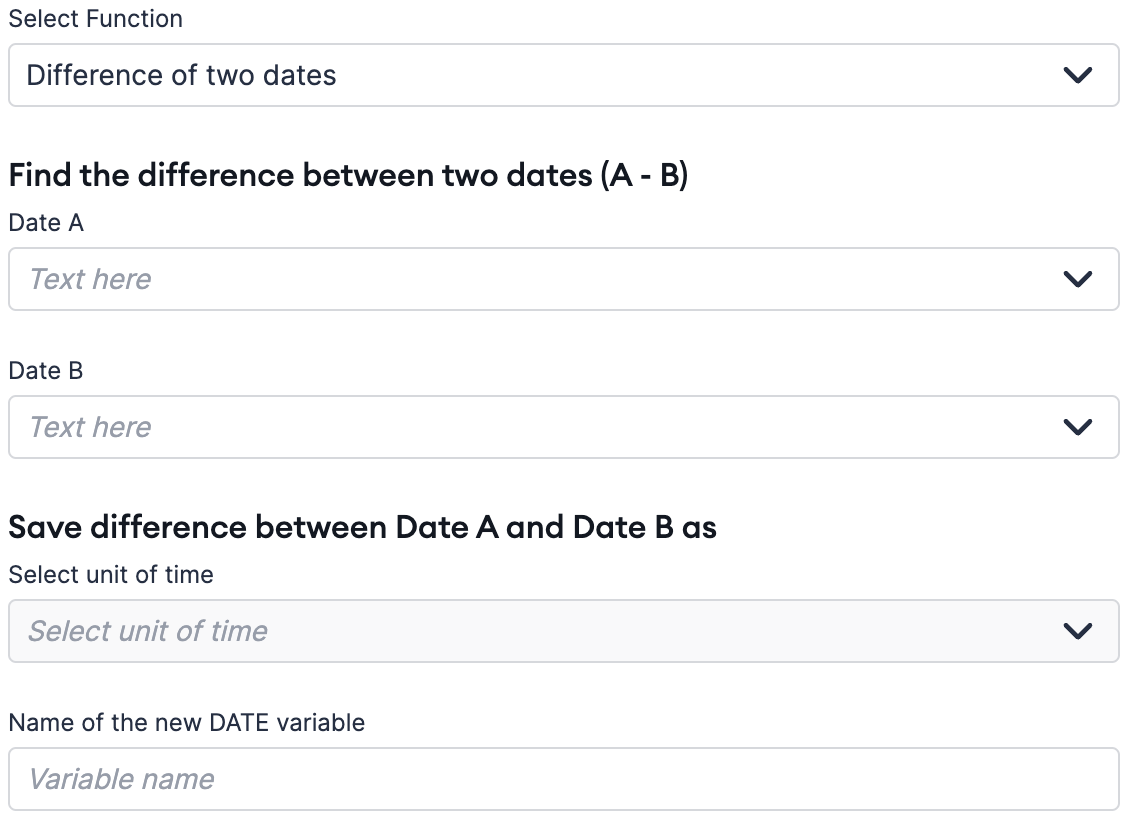# DATE_CALC Block

The Date Calculation block allows you to add and subtract time periods from a date variable as well as calculate the difference between two dates. With this block, you can easily perform complex date calculations without needing to write any code.

NOTE: The output date variable when adding or subtracting time periods will inherit the timezone of the original date variable.

To add time periods to a date variable, you can use the "Add time period to date" option. This will reveal additional form fields where you can specify the time period you want to add and the date variable you want to add it to. Time periods can be specified in minutes, hours, days, weeks, or months.You can then specify a name for the new or output date variable. This new date variable will inherit the timezone associated with the original date variable. This means you do not need to make your own timezone adjustments if your original date variable was already associated with the correct timezone. For reference, the date input on FORM blocks captures the end user's timezone.

Subtracting Time Periods

Similarly, to subtract time periods from a date variable, you can use the "Subtract time period from date" option. This will reveal additional form fields where you can specify the time period you want to subtract and the date variable you want to subtract it from. As with adding time periods, time periods can be specified in minutes, hours, days, weeks, or months.

Similarly, the new date variable will inherit the timezone associated with the original date variable.

Calculating the Difference Between Two Dates

To calculate the difference between two dates, you can use the "Difference of two dates" option. This will reveal additional form fields where you can specify the two dates you want to calculate the difference between. The result can be returned in minutes, hours, days, weeks, or months.Examples

Here are a few examples of how you can use the Date Calculation block:

• Freezing or locking assessments until a certain date - For example, preventing the end user from progressing an assessment until a specific date 20 days in the future.
• Conditionally routing end users based on the length of their relationship – In certain use cases, the length of a relationship determines the next steps for the end user. The Date Calculation block can be used to calculate the length of a relationship, which can then be used to conditionally route the end user.

If you have any questions or feedback, please don't hesitate to get in touch with our support team.